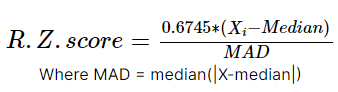# Robust Z-Score Method

Also known as the Median Absolute Deviation method, it is similar to Z-score method with some changes in parameters. Since mean and standard deviations are heavily influenced by outliers, instead of them we will be using median and absolute deviation from median.Suppose x follows a standard normal distribution. The MAD will converge to the median of the half normal distribution, which is the 75% percentile of a normal distribution, and N(0.75) is approximately equal to 0.6745.

INSTRUCTIONS
• First we will import `Numpy` as `np` and `scipy.stats` as `stats`

``````import numpy as <<your code goes here>>
import scipy.stats as <<your code goes here>>
``````
• Next, we will use the same datapoints we used previously

``````x = [5, 5, 5, -99, 5, 5, 5, 5, 5, 5, 88, 5, 5, 5]
``````
• Now, we will define a function `calculate_rzscore` that will detect the outliers using the robust z-score method

``````def <<your code goes here>>(data):
out=[]
med = np.median(data)
ma = stats.median_absolute_deviation(data)
for i in data:
z = (0.6745*(i-med))/ (np.median(ma))
if np.abs(z) > 3:
out.append(i)
print("Outliers:",out)
``````
• Finally, we will call the function using our datapoints

``````calculate_rzscore(<<your code goes here>>)
``````

No hints are availble for this assesment

Answer is not availble for this assesment

Note - Having trouble with the assessment engine? Follow the steps listed here